### Response Level Ordering

Response level ordering is important because, by default, PROC SURVEYLOGISTIC  models the probabilities of response levels with lower Ordered Values. Ordered Values, displayed in the "Response Profile" table, are assigned to response levels in ascending sorted order. That is, the lowest response level is assigned Ordered Value 1, the next lowest is assigned Ordered Value 2, and so on. For example, if your response variabletakes values in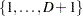, then the functions of the response probabilities modeled with the cumulative model are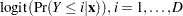and for the generalized logit model they are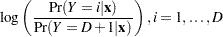where the highest Ordered Value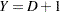is the reference level. You can change these default functions by specifying the EVENT=, REF=, DESCENDING, or ORDER= response variable options in the MODEL statement.

For binary response data with event and nonevent categories, the procedure models the function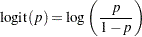whereis the probability of the response level assigned to Ordered Value 1 in the "Response Profiles" table. Since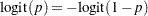the effect of reversing the order of the two response levels is to change the signs ofandin the model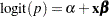.

If your event category has a higher Ordered Value than the nonevent category, the procedure models the nonevent probability. You can use response variable options to model the event probability. For example, suppose the binary response variable Y takes the values 1 and 0 for event and nonevent, respectively, and Exposure is the explanatory variable. By default, the procedure assigns Ordered Value 1 to response level Y=0, and Ordered Value 2 to response level Y=1. Therefore, the procedure models the probability of the nonevent (Ordered Value=1) category. To model the event probability, you can do the following:

• Explicitly state which response level is to be modeled by using the response variable option EVENT= in the MODEL statement:

```model Y(event='1') = Exposure;
```
• Specify the response variable option DESCENDING in the MODEL statement:

```model Y(descending)=Exposure;
```
• Specify the response variable option REF= in the MODEL statement as the nonevent category for the response variable. This option is most useful when you are fitting a generalized logit model.

```model Y(ref='0') = Exposure;
```
• Assign a format to Y such that the first formatted value (when the formatted values are put in sorted order) corresponds to the event. For this example, Y=1 is assigned formatted value 'event' and Y=0 is assigned formatted value 'nonevent.' Since ORDER= FORMATTED by default, Ordered Value 1 is assigned to response level Y=1 so the procedure models the event.

```proc format;
value Disease 1='event' 0='nonevent';
run;

proc surveylogistic;
format Y Disease.;
model Y=Exposure;
run;
```